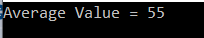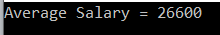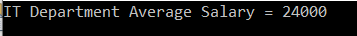# Linq Average Method in C# with Examples

In this article, I am going to discuss the Linq Average Method in C# with examples. Please read our previous article before proceeding to this article where we discussed the Linq Min Aggregate Function with some examples. As part of this article, we are going to discuss the following pointers.

1. What is Linq Average Method in C#?
2. Multiple examples using both Method and Query syntax.
##### What is Linq Average Method in C#?

The Linq Average method is used to calculate the average of numeric values from the collection on which it is applied. This Average method can return nullable or non-nullable decimal, float or double value. Let us understand the Average() method with some examples.

##### Example1:

The following example calculates the average value of all the integers present in the collection.

```using System;
using System.Linq;
namespace LINQDemo
{
class Program
{
static void Main(string[] args)
{
int[] intNumbers = new int[] { 60, 80, 50, 90, 10, 30, 70, 40, 20, 100 };

//Using Method Syntax
var MSAverageValue = intNumbers.Average();

//Using Query Syntax
var QSAverageValue = (from num in intNumbers
select num).Average();

Console.WriteLine("Average Value = " + MSAverageValue);
}
}
}
```

Output:Note: We don’t have any operator called Average in Linq to write the query syntax. So here we need to use the mixed syntax.

##### Example2: Linq Average Method with filter

Now we need to return the average value from the collection where the number is greater than 50.

```using System;
using System.Linq;
namespace LINQDemo
{
class Program
{
static void Main(string[] args)
{
int[] intNumbers = new int[] { 60, 80, 50, 90, 10, 30, 70, 40, 20, 100 };

//Using Method Syntax
var MSAverageValue = intNumbers.Where(num => num > 50).Average();

//Using Query Syntax
var QSAverageValue = (from num in intNumbers
where num > 50
select num).Average();

Console.WriteLine("Average Value = " + MSAverageValue);
}
}
}
```

It will print the output as 80.

##### Working with Complex Type:

We are going to work with the following Employee class.

```using System.Collections.Generic;
namespace LINQDemo
{
public class Employee
{
public int ID { get; set; }
public string Name { get; set; }
public int Salary { get; set; }
public string Department { get; set; }

public static List<Employee> GetAllEmployees()
{
List<Employee> listStudents = new List<Employee>()
{
new Employee{ID= 101,Name = "Preety", Salary = 10000, Department = "IT"},
new Employee{ID= 102,Name = "Priyanka", Salary = 15000, Department = "Sales"},
new Employee{ID= 103,Name = "James", Salary = 50000, Department = "Sales"},
new Employee{ID= 104,Name = "Hina", Salary = 20000, Department = "IT"},
new Employee{ID= 105,Name = "Anurag", Salary = 30000, Department = "IT"},
new Employee{ID= 106,Name = "Sara", Salary = 25000, Department = "IT"},
new Employee{ID= 107,Name = "Pranaya", Salary = 35000, Department = "IT"},
new Employee{ID= 108,Name = "Manoj", Salary = 11000, Department = "Sales"},
new Employee{ID= 109,Name = "Sam", Salary = 45000, Department = "Sales"},
new Employee{ID= 110,Name = "Saurav", Salary = 25000, Department = "Sales"}
};

return listStudents;
}
}
}```

We create the Employee class with four properties such as ID, Name, Salary, and Department along with the GetAllEmployees() which in turn going to return the list of all the employees.

##### Example3:

The following example returns the average salary of all the employees.

```using System;
using System.Linq;
namespace LINQDemo
{
class Program
{
static void Main(string[] args)
{
//Using Method Syntax
var MSAverageSalary = Employee.GetAllEmployees()
.Average(emp => emp.Salary);

//Using Query Syntax
var QSAverageSalary = (from emp in Employee.GetAllEmployees()
select emp).Average(e => e.Salary);

Console.WriteLine("Average Salary = " + MSAverageSalary);
}
}
}```

Output:##### Example4:

The following example calculates the Average Salary of the IT department.

```using System;
using System.Linq;
namespace LINQDemo
{
class Program
{
static void Main(string[] args)
{
//Using Method Syntax
var MSAverageSalary = Employee.GetAllEmployees()
.Where(emp => emp.Department == "IT")
.Average(emp => emp.Salary);

//Using Query Syntax
var QSAverageSalary = (from emp in Employee.GetAllEmployees()
where emp.Department == "IT"
select emp).Average(e => e.Salary);

Console.WriteLine("IT Department Average Salary = " + MSAverageSalary);